Select Page

Deep Learning and Machine Learning are no longer a novelty. Many applications are utilizing the power of these technologies for cheap predictions, object detection and various other purposes. At this blog, we usually write about deep learning, but we felt the need to address some more standard Machine Learning techniques and algorithms and go back to where it all started. In this article, we start off simple with Linear Regression. It is a well-known algorithm and it is the basics of this vast field. Linear Regression is, sort of, the root of it all. We will address theory and math behind it and show how we can implement this simple algorithm using several different technologies.

Are you afraid that AI might take your job? Make sure you are the one who is building it.

STAY RELEVANT IN THE RISING AI INDUSTRY! 🖖

For the purpose of this article, make sure that you have installed the following Python libraries:

• NumPy – Follow this guide if you need help with installation.
• SciKit Learn – Follow this guide if you need help with installation.
• TensorFlow – Follow this guide if you need help with installation.
• Pytorch – Follow this guide if you need help with installation.

Once installed make sure that you have imported all the necessary modules that are used in this tutorial.

``````import pandas as pd
import numpy as np
import matplotlib.pyplot as plt

from sklearn.linear_model import LinearRegression, SGDRegressor
from sklearn.metrics import mean_squared_error
from sklearn.model_selection import train_test_split
from sklearn.preprocessing import StandardScaler
from sklearn.pipeline import make_pipeline

import tensorflow as tf
import torch``````

Also, make sure that you are familiar with the basics of linear algebra, calculus and probability.

## Simple Linear Regression

Sometimes data that we have is quite simple. Sometimes, the output value of the dataset is just the linear combination of features in the input example. Let’s simplify it even further and say that we have only one feature in the input data. A mathematical model that describes such a relationship can be is presented with the formula:For example, let’s say that this is our data:In this particular case, the mathematical model that we want to create is just a linear function of the input feature, where b0 and b1 are the model’s parameters. These parameters should be learned during the training process. After that, the model should be able to give correct output predictions for new inputs. To sum it up, during training we need to learn b0 and b1 based on the values of x and y, so our f(xi) is able to return correct predictions for the new inputs. If we want to generalize even further we can say that model makes a prediction by adding a constant (bias term – b0) on the precomputed weighted sum (b1) of the input features. However, let’s back to our example and clear things up a little bit before we dive into generalization. Here is what the aforementioned data looks like on the plot:Our linear regression model, by calculating optimal b0 and b1, produces a line that will best fit this data. This line should be optimally distanced from all points in the graph. It is called the regression line. So, how does the algorithm calculates b0 and b1 values?In the formula above, f(xi) represents the predicted output value for ith example from the input, and b0 and b1 are regression coefficients that represent the y-intercept and slope of the regression line. We want that value to be as close as possible to the real value – y. Thus model needs to learn the values regression coefficients b0 and b1, based on which model will be able to predict the correct output. In order to make these estimates, the algorithm needs to know how bad are his current estimations of these coefficients. At the beginning of the training process, we feed samples into the algorithm which calculates output f(xi) of the current sample, based on initial values of regression coefficients. Then the error is calculated and coefficients are corrected. Error for each sample can be calculated like this:Meaning, we subtract estimated output from the real output. Note that this is a training process and we know the value of the output in the i-th sample. Because ei depends on coefficient values it can be described by the function. If we want to minimize ei and for that, we need to define a function based on which we will do so. In this article, we use the Least Squares Technique and define the function that we want to minimize as: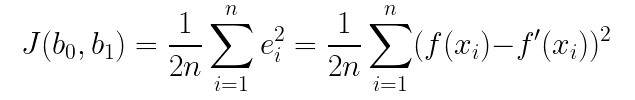The function that we want to minimize is called the objective function or loss function. In order to minimize ei, we need to find coefficients b0 and b1 for which J will hit the global minimum. Without going into mathematical details (you can check out that here), here is how we can calculate values for b0 and b1:Here SSxy is the sum of cross-deviations of y and x: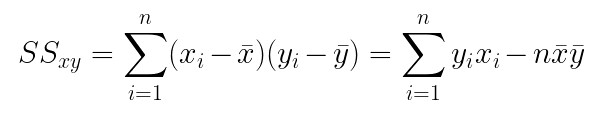while SSxx is the sum of squared deviations of x:Ok, so much for the theory, let’s implement this algorithm using Python.

## Simple Linear Regression Python Implementation

The algorithm we discussed previously is implemented withing SimpleLinearRegression class:

``````class LinearRegression():
''' Class that implemnets Simple Linear Regression '''
def __init__(self):
self.b0 = 0
self.b1 = 0

def fit(self, X, y):
mean_x = np.mean(X)
mean_y = np.mean(y)

SSxy = np.sum(np.multiply(X, y)) - len(x) * mean_x * mean_y
SSxx = np.sum(np.multiply(X, x)) - len(x) * mean_x * mean_x

self.b1 = SSxy / SSxx
self.b0 = mean_y - self.b1 * mean_x

def predict(self, input_data):
return self.b0 + self.b1 * input_data``````

This class has two functions, of which fit method is extremely interesting. In this method, we first calculate the mean values of input data and expected output. Then we calculate SSxy and SSxx and utilize those values to calculate b0  and b1. Basically, based on the input data we calculated necessary values for b0 and b1, ie. this is how we created our model. We can consider the fit method as our training function. The predict method uses these values to calculate values for the new data. Here is how we can use this function on the data from the previous chapter:

``````x = np.array([1, 2, 3, 4, 5, 6, 7, 8, 9, 10, 11])
y = np.array([6, 6, 11, 17, 16, 20, 23, 23, 29, 33, 39])

model = LinearRegression()
model.fit(x, y)``````

Then we use the trained model to predict new values and plot regression line:

``````predictions = model.predict(x)
plt.scatter(x = x, y = y, color='orange')
plt.plot(predictions, color='orange')
plt.show()``````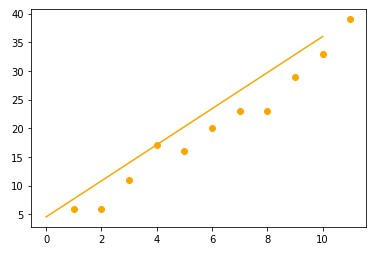## Multiple Linear Regression

Ok, that was super simple. The usage of this example is very limited since we usually end up with datasets with more features in them. Let’s take it up a notch and get a little more practical…and mathematical. We observe a set of labeled samples {(xi, yi)} Ni=1. The N is the size of the set, while xi is the D-dimensional feature vector and yi is the output. Every feature x is the real number. One such dataset is the famous Boston Housing Dataset. Once we load it with Pandas, we get something like this:

``````data = pd.read_csv('./data/boston_housing.csv')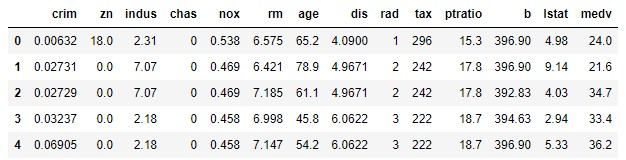In this dataset, the output is the medv feature, while the rest of the features are input features. As you can see there are several features (x1 – crim, x2-zn,….) for each sample i. Now, we can generalize principles of linear regression and use them on a dataset with more features. We can present the model with a formula: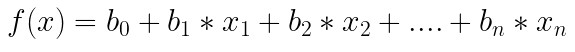Or to simplify it even further: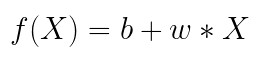We changed the notion there a little bit, but it is essentially the same as the previous formula, it is just vectorized. The bias b0 became b. The w is now a D-dimensional vector (because we have a D number of features, remember) of parameters. To predict the y for a given x we use this model. Obviously, we want to find the optimal values for coefficients (w, b) for which the model will output accurate predictions. Unlike simple linear regression which creates a line, multiple linear regression creates a hyperplane, since every feature represents one dimension. This hyperplane is chosen like that to be as close to all sample values as possible. To calculate the optimal coefficient, this time we want to minimize  Mean Squared Error function: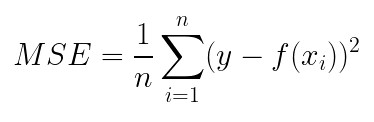To quickly find the values of w and b that minimize MSE we use the so-called Normal Equation. This equation gives direct results for mentioned coeficients:Ok, let’s utilize this in the code.

## Multiple Linear Regression Python Implementation

The algorithm we discussed previously is implemented withing MultipleLinearRegression class:

``````class MultipleLinearRegression():
''' Class that implements Multiple Linear Regression '''
def __init__(self):
self.b = 0
self.w = []

def fit(self, X, y):
# If there is only one feature we need to reshape input.
if len(X.shape) == 1:
X.reshape(-1, 1)

# Add 'ones' to model coefficient b in data.
ones = np.ones(shape=X.shape).reshape(-1, 1)
X = np.concatenate((ones, X), 1)

coeficients = np.linalg.inv(X.transpose().dot(X)).dot(X.transpose()).dot(y)
self.b = coeficients
self.w = coeficients[1:]

def predict(self, X):
predictions = []
for x in X:
prediction = self.b

for xi, wi in zip(x, self.w):
prediction += wi * xi

predictions.append(prediction)

return predictions``````

The ‘blueprint‘ is the same as for the previous implementation, we have two functions fit and predict. The first one creates the model, while the other utilizes it. In the fit method, we first reshape input data if it has only one dimension. This way we incorporate the implementation of Simple Linear Regression in this class as well.  Then we extend input data with a vector of ones. This is done because the bias coefficient – b is not implicitly modeled in data, i.e. this value is not multiplied with any feature from the input data so we need to add it manually. So, we add an array of ones so this value is calculated as well. After that, we utilize the Normal Equation and calculate all the coefficients. Here is how we can use this class on the Boston Housing Dataset:

``````X = data.drop('medv', axis=1).values
y = data['medv'].values

model = MultipleLinearRegression()
model.fit(X, y)

predictions = model.predict(X)``````

First, we separate input data – X from the output data – y. Then we create an object of MultipleLinearRegression class and call fit method with input data. Finally, we call the predict method to generate predictions. To compare predictions made by our implementation with the actual values, we can create Pandas Dataframe:

``````predictions = model.predict(X)
pd.DataFrame({
'Actual Value': y,
'Vanilla Model Prediction': predictions,
})``````From the results, we can see that overall results are close but not too close to the real results. Keep in mind that we haven’t performed any data preprocessing and that we haven’t scaled the data. Let’s see how we can do that and use SciKit Learn implementation of the same algorithm.

## Using SciKit Learn

Our previous implementations are quite vanilla. If we try to use them on a large dataset we may encounter some problems. For starters, it loads all the data, so we can only hope that we have enough memory for the whole dataset. Apart from that, this is really computing expensive ways to perform Linear Regression. The computational complexity of inverting a matrix of n features (which normal equation does) is typically about O(n2.4) to O(n3). This means that if we have a larger dataset, which has twice as many features (2*n) computation time will be 5.3 to 8 times larger.We haven’t supported gradient descent or some other smart optimization technique. Lucky for us, guys from SciKit Learn did. SciKit Learn is a great tool for machine learning in Python. Using it we can not only use algorithms out of the box but preprocess data as well. So let’s first fix several things we overlooked in the previous implementation. We need to split our data into training and testing sets, this is mandatory. Apart from this, we can compare the results of our implementation with the out-of-the-box solution that SciKit Learn provides.

``````# Split the data
X_train, X_test, y_train, y_test = train_test_split(X, y, test_size=0.2, random_state=33)

# Re-Train implementation
model.fit(X_train, y_train)

# Use and train SciKit Learn Linear Regression
sk_model = LinearRegression()
sk_model.fit(X_train, y_train)

# Make predictions
sk_predictions = sk_model.predict(X_test)
predictions = model.predict(X_test)

# Compare
pd.DataFrame({
'Actual Value': y_test,
'Vanilla Model Prediction': predictions,
'SciKit Model Prediction': sk_predictions,
})``````

Here is the output:We can see that we have the same results using both implementations. Also, we can see that results are not that bad. Can we improve them? We can try using SGDRegressor. This is a Linear Regression Model that uses Stochastic Gradient Descent for the optimization. If we want to use this algorithm we must scale data. For that purpose we utilize SciKit Learn’s Pipeline, StandardScaler and SGDRegressior:

``````sgd_pipeline = make_pipeline(StandardScaler(), SGDRegressor(max_iter=10000, alpha=0.1))
sgd_pipeline.fit(X_train, y_train)

sgd_predictions = sgd_pipeline.predict(X_test)

pd.DataFrame({
'Actual Value': y_test,
'Vanilla Model Prediction': predictions,
'SciKit Model Prediction': sk_predictions,
'SGD Model Prediction': sgd_predictions,
})``````

First, we create pipeline of StandardScalerI and SGDRegressor. This means that when we use fit method of this pipeline, data will first be processed by the scaler and then regressor with be trained. Here is the comparison of the results:We can see that results are mixed. Sometimes we get better results using the standard approach, while others we get better results using Stochastic Gradient Descent.

## Using Deep Learning Frameworks

Alternatively, we may want to pick some deep learning frameworks for the implementation of Linear Regression with Stochastic Gradient Descent. In this article, we use TensorFlow and PyTorch. Note that we are not using neural networks, but we use these frameworks to implement Linear Regression from scratch. Thus it is making this part of the article more of a thought exercise than something you would regularly do. If you want to get more familiar with TensorFlow take a look at this article, while if you want to learn more about PyTorch check out this mini-series of articles.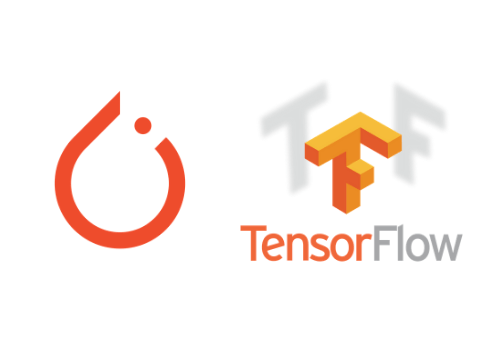### Using TensorFlow

So far we got a pretty clear idea of how the classes should look like. Here is implementation of Linear Regression with Stochastic Gradient Descent with TensorFlowTensorFlowLinearRegression:

``````class TensorFlowLinearRegression:
''' Class that implemnets Multiple Linear Regression with TensorFlow'''
def __init__(self, num_of_features):
self.var = 0.

self.w = tf.Variable(1., shape=tf.TensorShape(None))
self.w.assign([self.var] * num_of_features)

self.b = tf.Variable(self.var)
self.learning_rate = 0.000001

def _mse(self, true, predicted):
return tf.reduce_mean(tf.square(true-predicted))

def fit(self, X, y, epochs=5):

if len(X.shape)==1:
X=tf.reshape(X,[X.shape,1])

for i in range(epochs):
loss = self._mse(y, self.predict(X))

if i % 1000 == 0:
print(f'Epoch: {i} - Loss: {loss}')

self.w.assign_sub(self.learning_rate * dw)
self.b.assign_sub(self.learning_rate * db)

def predict(self, X):
return tf.reduce_sum(self.w * X, 1) + self.b``````

This is really similar to our initial implementation of Linear Regression, but it uses gradient descent. The core of the functionality is, of course, in the fit method. Notice that for this algorithm we need to define the number of epochs. When we push our complete dataset through the algorithm we have performed one epoch. In our implementation, we use GradientTape where we record operations for automatic differentiation. We calculate loss, for which we utilize the implementation of MSE with TensorFlow. Then we calculate gradients for w and b based on that loss. Finally, we align w and b. Here is how this class is used with Boston Household Dataset and the results we get once we use it:

``````X_train, X_test, y_train, y_test = train_test_split(X, y, test_size=0.2, random_state=33)

tf_model = TensorFlowLinearRegression(X_train.shape)
tf_model.fit(X_train, y_train, 10000)

tf_predictions = tf_model.predict(X_test)
pd.DataFrame({
'Actual Value': y_test,
'Vanilla Model Prediction': predictions,
'TensorFlow Prediction': tf_predictions,
})``````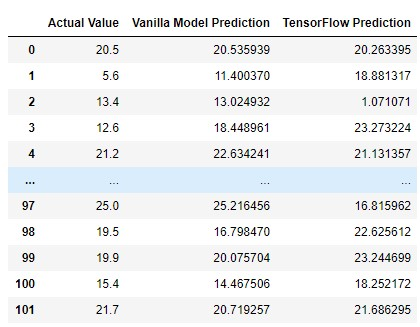As in the previous example, results are mixed, but it seems that vanilla implementation is somewhat better.### Using PyTorch

Ok, let’s do the completely same thing with PyTorch. This implementation is done in PyTorchLinearRegression:

``````class PyTorchLinearRegression:
''' Class that implemnets Multiple Linear Regression with PyTorch'''
def __init__(self, num_of_features):
self.learning_rate = 0.000001

def _model(self, X):
return X @ self.w.t() + self.b

def _mse(self, pred, real):
difference = pred - real

def fit(self, X, y, epochs):
X = torch.from_numpy(X).float()
y = torch.from_numpy(y).float()

for i in range(epochs):
predictions = self._model(X)
loss = self._mse(predictions, y)

if i % 1000 == 0:
print(f'Epoch: {i} - Loss: {loss}')

loss.backward()

def predict(self, X):
X = torch.from_numpy(X).float()
return self._model(X)``````

As you can notice, everything is completely the same except that we used PyTorch. The main difference we can see when we run this code. You will notice that PyTorch performs this process faster. Usage is the same as for the previous classes:

``````X_train, X_test, y_train, y_test = train_test_split(X, y, test_size=0.2, random_state=33)

torch_model = PyTorchLinearRegression(X_train.shape)
torch_model.fit(X_train, y_train, 10000)

torch_predictions = torch_model.predict(X_test)
torch_predictions = torch_predictions.detach().numpy()
pd.DataFrame({
'Actual Value': y_test,
'Vanilla Model Prediction': predictions,
'Torch Prediction': torch_predictions,
})``````## Conclusion

In this article, we had a chance to see what is Linear Regression and how it is performed. We could see how we can implement our own algorithm or use the one that is available in SciKit Learn. Apart from that, we could see how one can implement this algorithm with Stochastic Gradient Descent and deep learning frameworks.#### Nikola M. Zivkovic

CAIO at Rubik's Code

Nikola M. Zivkovic a CAIO at Rubik’s Code and the author of book “Deep Learning for Programmers“. He is loves knowledge sharing, and he is experienced speaker. You can find him speaking at meetups, conferences and as a guest lecturer at the University of Novi Sad.

Rubik’s Code is a boutique data science and software service company with more than 10 years of experience in Machine Learning, Artificial Intelligence & Software development. Check out the services we provide.

Ultimate Guide to Machine Learning with Python

Everything from Python basics to the deployment of Machine Learning algorithms to production in one place.

Become a Machine Learning Superhero TODAY!